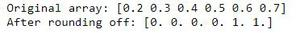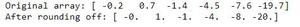# How to round elements of the NumPy array to the nearest integer?

• Last Updated : 08 Jun, 2022

Prerequisites: Python NumPy

In this article, let’s discuss how to round elements of the NumPy array to the nearest integer. numpy.rint() function of Python that can convert the elements of an array to the nearest integer.

Syntax: numpy.rint(x, /, out=None, *, where=True, casting=’same_kind’, order=’K’, dtype=None, subok=True[, signature, extobj]) = <ufunc ‘rint’>

Example 1:

## Python3

 `import` `numpy as n` `# create array``y ``=` `n.array([``0.2``, ``0.3``, ``0.4``, ``0.5``, ``0.6``, ``0.7``])``print``(``"Original array:"``, end``=``" "``)``print``(y)` `# round to nearest integer``y ``=` `n.rint(y)``print``(``"After rounding off:"``, end``=``" "``)``print``(y)`

Output:Example 2:

## Python3

 `import` `numpy as n` `# create array``y ``=` `n.array([``-``0.2``, ``0.7``, ``-``1.4``, ``-``4.5``, ``-``7.6``, ``-``19.7``])``print``(``"Original array:"``, end``=``" "``)``print``(y)` `# round to nearest integer``y ``=` `n.rint(y)``print``(``"After rounding off:"``, end``=``" "``)``print``(y)`

Output:My Personal Notes arrow_drop_up